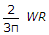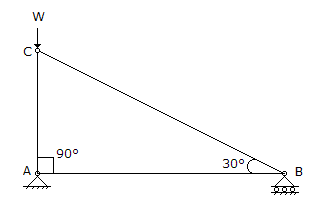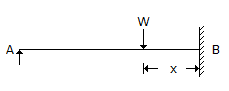# Civil Engineering - UPSC Civil Service Exam Questions

41.

The slenderness ratio of lacing bars should not exceed :

 A. 100 B. 200 C. 145 D. 180

Explanation:

No answer description available for this question. Let us discuss.

42.

A three-hinged semicircular arch of radius R carries a uniformly distributed load W per unit run over the whole span. The horizontal thrust is

 A. WR B. WR/2 C. 4/3π WR D.Explanation:

No answer description available for this question. Let us discuss.

43.

The acceleration components of a fluid particle are denoted as
1. local tangential acceleration.
2. convective tangential acceleration.
3. local normal acceleration.
4. convective normal acceleration.
In a curved nozzle fitted to the end of straight pipeline carrying water under variable head, the acceleration component that are present would include

 A. 1 and 2 B. 3 and 4 C. 1, 2 and 4 D. 1, 2, 3 and 4

Explanation:

No answer description available for this question. Let us discuss.

44.

In the truss shown in the given figure, the forces in the members AB and BC will be respectively (plus denotes tension)A. zero and zero B. - W/cos 60° and + W tan 60° C. - W/cos 60° and zero D. zero and + W tan 60°

Explanation:

No answer description available for this question. Let us discuss.

45.

Consider the following statements :
In the beam shown in the given figure, for all positions of load W (except x = 0)
1. bending moment is maximum at B
2. bending moment is maximum under load
3. deflection is zero at A
4. deflection is zero at B
Of these statements :A. 1 and 3 are correct B. 2 and 4 are correct C. 1 and 4 are correct D. 1, 3 and 4 are correct